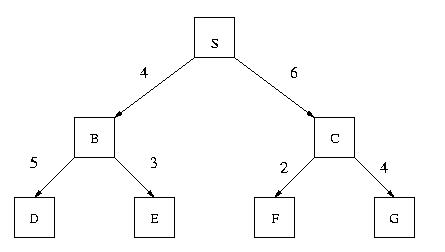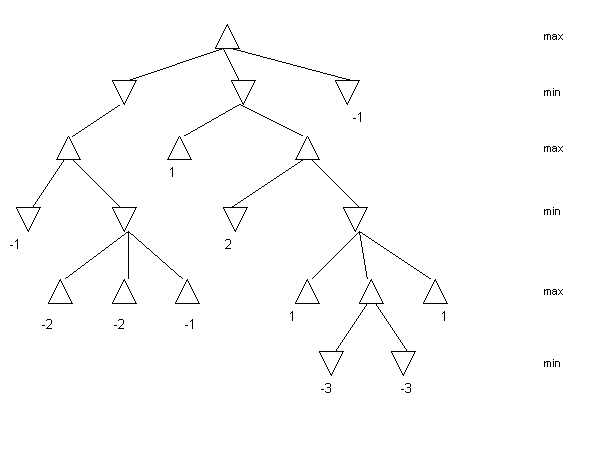## CS4341 Introduction to Artificial Intelligence. C2000 Exam 1 - February 4, 2000

### Prof. Carolina RuizDepartment of Computer ScienceWorcester Polytechnic Institute

#### Instructions

• Write your name on each page
• Ask in case of doubt

#### Problem I. Search (40 points)

Suppose that you need to find a path between S and G in the following graph. The number attached to each edge in the graph represents the COST of traversing the edge.Assume also that the ESTIMATED distances to the goal are given by the following table:

 Node: S B C D E F G Estimated Distance to G: 6 1 3 2 5 4 0

For EACH of the following search methods list the nodes in the order in which they are EXPANDED by the search method while looking for a solution. As an illustration, the solution for Depth 1st search is provided below.

1. Depth 1st Search

List: [S, B, D, E, C, F, G]

2. (5 points) Breadth 1st Search

List:

3. (5 points) Depth-limited Search (depth-limit = 2)

List:

4. (5 points) Iterative Deepening Search

List for depth-limit = 0:

List for depth-limit = 1:

List for depth-limit = 2:

List for depth-limit = 3:

5. (5 points) Branch-and-Bound (= Uniform Cost Search)

List:

6. (5 points) Greedy Search (= Best 1st Search)

List:

7. (5 points) A*

List:

8. (5 points) Hill-climbing

List:

9. (5 points) Beam Search (m = 2)

List:

#### Problem II. Game Playing (20 points + 10 EXTRA points)

Suppose you and a friend of yours are playing a board game. It is your turn to move, and the tree below represents your situation. The values of the evaluation function at the leaf nodes are shown in the tree. Note that in this tree not all leaf nodes are at the same level.

1. (20 points) Use the minimax procedure to select your next move. Show your work on the tree below.2. (10 EXTRA points) Use the minimax procedure TOGETHER WITH ALPHA-BETA PRUNING to select your next move. Mark with an "X" the nodes that don't need to be evaluated. Show your work on the tree below and explain your answer.#### Problem III. Rule-Based Systems (20 points)

Consider the following set of rules that describe when a person can vote in a presidential election.

• R1: IF ?x was born in the US THEN ?x is an American

• R2: IF ?x received US citizenship THEN ?x is an American

• R3: IF ?x's age >= 18 THEN ?x is an adult

• R4: IF ?x is American AND ?x is an adult THEN ?x can vote

Assume that the operator ">=" (greater than or equal) is a basic operator implemented in the inference engine.
The working memory contains the following assertions:

• A1: Bill's age is 16.

• A2: Sue received US citizenship.

• A3: Bill was born in the US.

• A4: Sue's age is 20.
Solve the following problems. Show your work.

1. (10 points) Use backward chaining to determine whether or not Bill can vote. Construct a tree showing the steps followed by backward chaining and show when and how the working memory is updated during the depth 1st search.

2. (10 points) Use forward chaining to derive ALL assertions that are derivable from this knowledge base (that is, from the set of rules together with the assertions in the working memory). Construct trees showing the steps followed by forward chaining and show when and how the working memory is updated during the process.

#### Problem IV. Frame Systems (20 points)

Consider the following hierarchy of frames.
```                   COMPUTER USERS
/           |
/            |
ako/             | ako
/              |
/               |
UNIX USERS             PC USERS           MICROSOFT
CUSTOMERS
\           /        \           /
\         /          \         /
ako\       /ako      ako\       /ako
\     /              \     /
\   /                \   /
LINUX USERS            WINDOWS98
USERS
\           /
\         /
is-a \       /is-a
\     /
\   /
AMY
```

1. (15 points) Give the class-precedence list for Amy that would be obtained by applying the topological-sorting algorithm to the previous graph. (You don't need to show the steps of the topological sorting algorithm.)

2. (5 points) Suppose that each of the classes Unix users, PC users and Computer Users contains a favorite programming language slot. The default value for this slot is:
• Fortran, for the Computer Users class.
• C, for the Unix Users class.
• C++, for the PC Users class.

What is the value obtained for Amy's favorite programming language according to the class-precedence list you constructed above? Explain you answer.